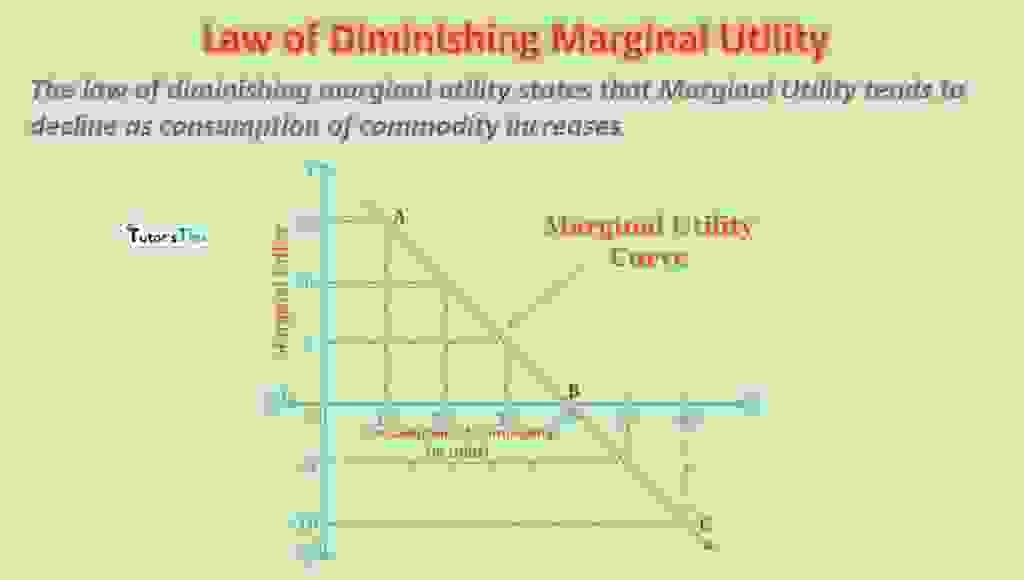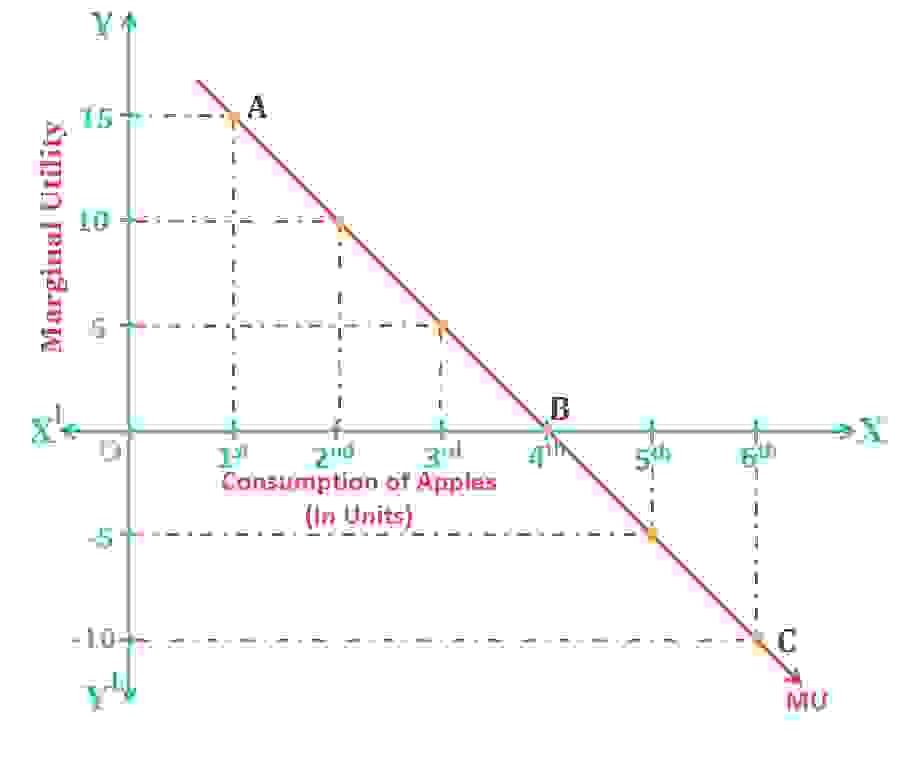# Law of Diminishing Marginal UtilityThe law of diminishing marginal utility states that Marginal utility tends to decline as consumption of commodity increases.

## Meaning of Marginal Utility :

It refers to the additional utility derived from the consumption of one more unit of a commodity in a given period.

MUn = TUn– TUn-1

Here,

MU denotes marginal utility from the nth unit

TUn denotes total utility from the nth unit

TUn-1 denotes total utility from the (n-1)th unit

In simple words, MU is the change in total utility when more unit of a given commodity is consumed.

Therefore,

“MU= Change in TU/ Change in units consumed”

Subscribe our Youtube Channel

## Types of Marginal Utility :

1. Positive Marginal Utility
2. Zero Marginal Utility
3. Negative Marginal Utility

### 1. Positive Marginal Utility:

When the total utility increases with the increase in consumption of additional units of a commodity, it is known as a positive marginal utility.

### 2. Zero Marginal Utility :

When total utility doesn’t change with the increase in consumption of additional units of a commodity, it is known as Zero marginal utility.

### 3. Negative Marginal Utility:

When the total utility decreases with the increase in consumption of additional units of a commodity, it is known as a negative marginal utility.

## Law of Diminishing Marginal Utility :

The law of diminishing marginal utility states that as more and more units of a commodity are consumed, the marginal utility derived from consuming additional units keeps on declining. It is also known as ‘Fundamental Law of Satisfaction’ or ‘Fundamental Psychological Law’ as it happens in respect of all goods and services. This concept is used to determine the quantity of a commodity that a consumer is willing to buy.

For Example,

Suppose, a consumer is hungry and buys a  burger to sate his hungriness, the burger will give him great satisfaction at first. After consuming one burger, he wouldn’t like to have another as his want is already satisfied with one burger. This is the case with most of the commodities.

### Definitions:

#### According to Marshall,

“The additional benefit which a person derives from a given stock of a thing diminishes with every increase in the stock that he already has.”

#### According to Chapman,

“The more we have of a thing, the less we want additional increments of it or the more we want not to have additional increments of it.”

#### In words of Prof.Boulding,

“As a consumer increases the consumption of any one commodity, keeping constant the consumption of all other commodities, the marginal utility of the variable commodity must eventually decline.”

### Assumptions of Law of Diminishing Marginal Utility :

1. Cardinal Measurement of Utility: This assumption states that utility should be expressed in cardinal numbers. For example, the utility derived from 1 unit of commodity ‘X’  is 10 units whereas the utility derived by consuming a unit of commodity ‘Y’ is 8 units. It eases the comparison of satisfaction derived from different commodities.
2. Constant Marginal Utility of Money: It is assumed that the marginal utility of money remains constant while spending money to consume the goods or services for satisfaction.
3. Independent Utility: This theory assumes that the total utility derived from a bundle of goods is the total of utility derived from individual commodities.

## Illustration of Law of Diminishing Marginal Utility  :

The following table shows the consumption pattern and utility derived by consuming each unit of apples :

 Units of Apples T. U. M. U. 1st 15 15(15-0) 2nd 25 10(25-15) 3rd 30 5 (30-25) 4th 30 0 (30-30) 5th 25 -5(25-30) 6th 15 – 10(15-25)

When the customer consumes the 1st unit of apple, it gives him the total utility and the marginal utility of 15 units. As he increases the consumption with the 2nd unit, the total utility increases to 25 units but the marginal utility declines to 10 units. Similarly, while consuming more units of apples, the total utility goes on increasing with diminishing rate. But after the consumption of the 3rd unit, the total utility stabilizes there as he gets maximum satisfaction there. As long as the marginal utility is positive, the total utility increases. When MU becomes zero, the TU remains constant shown by consumption of the 4th unit. After that point, the TU starts declining and MU becomes negative.

### Graphical Representation:Law of Marginal Utility

In fig, X-axis shows the units of apples consumed and Y-axis shows the marginal utility derived. MU is the marginal utility curve. It slopes downward from left to right indicating that 1st apple yields 15 utils,2nd 10 utils and 3rd 5 utils of marginal utility. 4th apple yields zero marginal utility. So, MU curve touches X-axis at point B. Consumption of next apple yields negative marginal utility and so, Marginal utility curve goes below X-axis. The point where MU is zero is known as a point of saturation.

### Relationship between TU and MU :

1. Total utility is equal to the sum of marginal utilities.
2. When the marginal utility is positive, the total utility increases.
3. The total utility is maximum when the marginal utility is zero,
4.  When the total utility starts declining, the marginal utility becomes negative.

### Limitations of Law of Marginal Utility :

1. Homogenous units of commodities: The different units of a commodity are not the same in all respects. The preferences of customers change with a period.
2. Standard Measurement of Consumption: The consumption of goods are measured in standard units. These units are variate and cannot be compared.
3. Continous Consumption: There is continuous consumption of goods and services. The units are consumed continuously one after another which is not reasonable.
4. Not applicable for luxury goods:  This law doesn’t applicable to luxurious goods like jewellery, fashionable clothes and luxurious cars and houses.
5. Related Goods:  The consumption of commodities are affected by the availability of related goods. In the absence of related goods, consumption and utility diminish.
6.  Cardinal Measurement is not possible: This theory assumes that the utility is measured in cardinal numbers, which is not correct.

Thanks Please share with your friends

Comment if you have any question.

References:

Introductory Microeconomics – Class 11 – CBSE (2020-21)